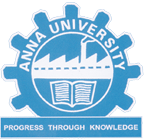MA5160 APPLIED PROBABILITY AND STATISTICS SYLLABUS - ANNA UNIVERSITY PG REGULATION 2017 - Anna University Internal marks 2018
OBJECTIVES:
This course is designed to provide the solid foundation on topics in applied probability and various statistical methods which form the basis for many other areas in the mathematical sciences including statistics, modern optimization methods and risk modeling. It is framed to address the issues and the principles of estimation theory, testing of hypothesis and multivariate analysis.MA5160 APPLIED PROBABILITY AND STATISTICS SYLLABUS

UNIT I PROBABILITY AND RANDOM VARIABLES
Probability – Axioms of probability – Conditional probability – Baye‘s theorem - Random variables - Probability function – Moments – Moment generating functions and their properties – Binomial, Poisson, Geometric, Uniform, Exponential, Gamma and Normal distributions – Function of a random  variable.

UNIT II TWO DIMENSIONAL RANDOM VARIABLES
Joint distributions – Marginal and conditional distributions – Functions of two dimensional random variables – Regression curve – Correlation.

UNIT III ESTIMATION THEORY
Unbiased estimators – Method of moments – Maximum likelihood estimation - Curve fitting by principle of least squares – Regression lines.

UNIT IV TESTING OF HYPOTHESIS
Sampling distributions – Type I and Type II errors – Small and large samples – Tests based on Normal, t, Chi square and F distributions for testing of mean, variance and proportions – Tests for independence of attributes and goodness of fit.

UNIT V MULTIVARIATE ANALYSIS
Random vectors and matrices – Mean vectors and covariance matrices – Multivariate normal density and its properties – Principal components - Population principal components – Principal components from standardized variables

TOTAL: 60 PERIODS

OUTCOMES:

After completing this course, students should demonstrate competency in the following topics:
• Basic probability axioms and rules and the moments of discrete and continuous random variables.
• Consistency, efficiency and unbiasedness of estimators, method of  maximum likelihood estimation and Central Limit Theorem.
• Use statistical tests in testing hypotheses on data.
• Perform exploratory analysis of multivariate data, such as multivariate normal density, calculating descriptive statistics, testing for multivariate normality.
• The students should have the ability to use the appropriate and relevant, fundamental and applied mathematical and statistical knowledge, methodologies and modern computational tools.
REFERENCES:
1. Devore, J. L., ―Probability and Statistics for Engineering and the Sciences‖, 8th Edition, Cengage Learning, 2014.
2. Dallas E. Johnson, ―Applied Multivariate Methods for Data Analysis‖, Thomson and Duxbury press, 1998.
3. Gupta S.C. and Kapoor V.K.,‖ Fundamentals of Mathematical Statistics‖, Sultan and Sons, New Delhi, 2001.
4. Johnson, R.A., Miller, I and Freund J., "Miller and Freund‘s Probability and Statistics for Engineers ", Pearson Education, Asia, 8th Edition, 2015.
5. Richard A. Johnson and Dean W. Wichern, ―Applied Multivariate Statistical Analysis‖, 5th Edition, Pearson Education, Asia, 2002.

Anna University PG Regulation 2017 CSE Syllabus, Ma5160 Applied Probability And Statistics Syllabus, Reg 2017 Ma5160 Syllabus, 1st Sem PG Maths 1